# Online Math Problem Solver

TutorBin CalculatorMatrix algebraCalculusLinear SystemVisualizationSimplificationUnit ConversationSimple calculator

Math Problem Solver by TutorBin is any student’s best friend when it comes to solving Algebra problems. This step by step math problem solver is your solution to solving some of the most comprehensive math questions across algebra, calculus, linear systems, quadratic equations, etc. By providing all the necessary tools, we are one of the top problem solving resources available for students, parents, and teachers.

The aim and goal of Math Problem Solver by TutorBin is simple. We aspire to bring math-assistance to all students to help improve their grades in a seamless manner. We intend to augment and increase the functionalities over time to make solving math problems even more easy.

As a free math problem solver, it is our endeavour to help students get step by step solutions and get a strong understanding on their basics. It is a part of our larger vision for TutorBin where we aim to offer high quality homework help to all students to accelerate their academic performance. If you are looking for step by step solutions, click here and get access to our network of global expert tutors to help you out.

\$
Years
months
%

Formula

Monthly payment = P i (1+i) ^ n / (1+i) ^ n - 1
P - the principal, or the initial borrowed amount
i - interest rate per repayment period
n - number of payments over the life of the mortgage

Supported Operators
Trigonometric Functions
• Trigonometric - sin(x), cos(x), tan(x), cot(x), sec(x), csc(x)
• Hyperbolic Trigonometric - sinh(x), cosh(x), tanh(x), coth(x), sech(x), csch(x)
• Inverse Trigonometric - asin(x), acos(x), atan(x), acot(x), asec(x), acsc(x)
Arithmetic Operations
• Addition : " + ",   e.g. 5+x
• Subtraction: " - ",  e.g. 2-x
• Multiplication: " * ",  e.g. 2*x
• Division: " / ",  e.g. 1/x
• Numeric Fractions: Rational(a,b) = a/b
Exponential and Logarithms
• Exponential : e = exp(1), e5 = exp(5)
• Logarithms : ln(x) = log(x,base = exp(1)), log10(x) = log(x,base = 10)
Powers
• Rating a number to a power could be achieved via '', e.g. x2 is interpreted as x2, and "sqrt(x)" as &Sqrt; x
Complex Numbers
• Complex number are inserted as follows: 1i = i, 2i = 2i, Where i = &Sqrt; -1. Note that we do not need the multiplication sign.
Solved Examples
Matrix Algebra

A matrix is an array of numbers , several operations can be performed on matrices.

Adds elements that are in matching positions and the two matrices must be the same size.

Example 1 - Add the following 2×2 dimension matrices :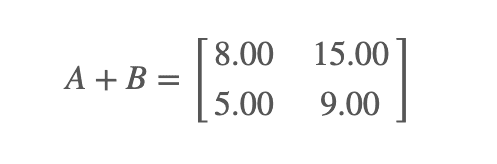Subtraction :

Subtracts elements that are at matching positions and the two matrices must be the same size.

Example 1 - Subtract the following 2×2 dimension matrices :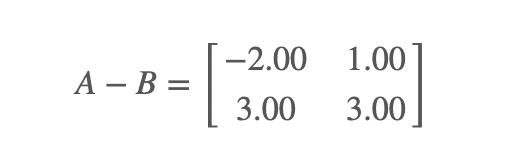Multiplication :

For matrix multiplication, the number of rows of the first matrix must be equal to the number of columns of the second matrix.

Example 1 - Multiply the following 2×2 dimension matrices :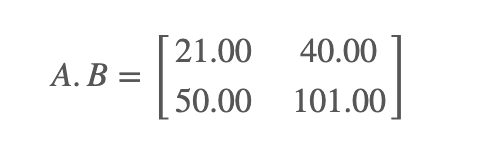Transpose :

Swapping the elements of columns and rows.

Example 1 - Find the transpose of the 2x3 matrix below :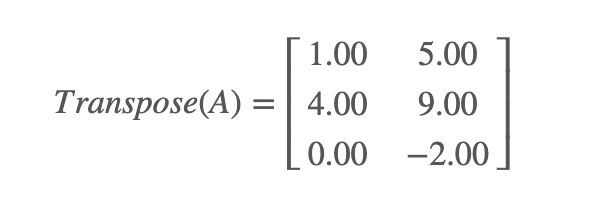Determinant :

It can be calculated only for square matrix.

Example 1 - For 2X2 matrix :Inverse :

Just like a reciprocal of a number is called its inverse , similarly the reciprocal of a matrix is the inverse of a matrix.

Example 1 - Find the inverse of the 2x2 matrix below :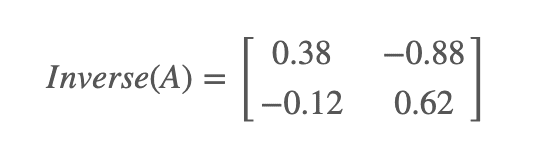Prerequisite and fundaments

A matrix is a rectangular array of numbers, symbols, and expressions arranged in rows and columns.The size of a matrix is defined by the number of rows and columns in it. If a matrix contains m rows and n columns then it is said to be a matric of m x n size.

A matrix with the same number of rows and columns is called asquare matrix.

While performing addition or subtraction, add/subtract each element in the first matrix with the corresponding element in the second matrix. Addition and subtraction can be only performed between the matrices of the same size and the resultant matrix is also of the same size .

While Multiplying two matrices the elements of rows in the first matrix are multiplied with the corresponding columns in the second matrix. The first element of the first matrix is multiplied with each element of first column of the second matrix , the summation becomes the first entry of the resultant column, in the same way, we can find the product of two matrices.

Multiplication can be performed between the two matrices only if the no. of columns in the first matrix is equal to the no. of rows in the second matrix. If the dimensions of the first matrix are AxB and the dimensions of the second matrix are BxC then the resultant matrix would be AxC.

An identity matrix is a diagonal matrix in which all the diagonal elements are 1 and all the remaining elements are 0.

Calculus is a branch of mathematics that studies continuous change,it is basically used to study or work with very small quantities. Calculus is used to gain more understanding of the nature of space, time and motion.

The two main branches of Calculus are:

• Differential calculus: It differentiates something into small pieces to study how it changes.It is the study of definitions, properties and, applications of derivatives of a function, and the process of finding derivatives is called differentiation.

The equation representing the relationship between the quantity that continuously varying concerning in another quantity.

Eg. f(x)=dy/dx is a differential equation.

Order of differential equation: Order of the highest order derivative present in the differential equation.

Degree of Differential equation: The power of highest order derivative is the degree of differential equation.

• Integral Calculus: Integral calculus integrates small pieces to find the whole.Integrals are of two types:1. Definite Integral2. Indefinite Integral.Integration is the process of finding the antiderivative.Eg. xndx = x n+1/n+1 + cC is called the integration constant.

A Linear system is nothing but the collection of linear equations involving the same set of variables. The solution of a linear system is the values of variables that satisfy all the linear equations. You have to enter the coefficients of variables in a matrix and constants in another matrix, our calculator will deliver the results you were looking for.

All the linear equations in a linear system have same set of variables of order one and each equation forms a straight line on the graphs.

Any equation with degree 2 is a quadratic equation . A quadratic equation is in the form ofax2+bx+c=0, the solution of a quadratic equation is given by,x = ( -b ± √ b2-4ac ) / 2a.

These solutions can be given in the form of real numbers or complex numbers.

Visualization is used to represent the given equations on the graphs or 2d plane.A linear equation always forms a straight line on the graph whereas a quadratic equation with real solutions forms a curve.

To solve an equation it is important to simplify it to write it in the simplest form.Different methods for simplifying an expression are:

• Rationalize
• Factoring
• Expansion
• Combine like terms
• Clear out fractions by multiplying,etc.